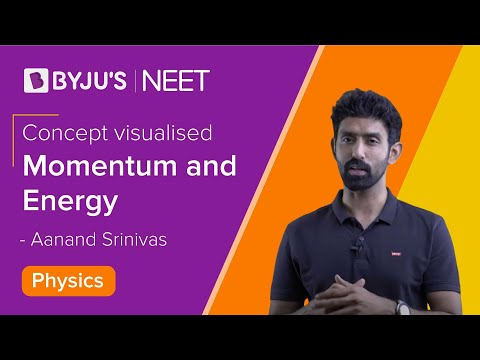# Relation Between Kinetic Energy and Momentum

## Kinetic Energy

The kinetic energy of an object is the energy associated with the object which is under motion. It is defined as “the energy required by a body to accelerate from rest to stated velocity.” It is a vector quantity.

### Kinetic Energy formula

Mathematically expressed as-

$$K.E=\frac{1}{2}mv^{2}$$

Where,

m is the mass of the object measured in kg.

v is the velocity of the object measured in m/s

### Kinetic Energy unit

The SI Unit of Kinetic Energy is Joules.

## Momentum

The momentum of an object is the virtue of its mass. It is defined as the product of mass and velocity. It is a vector quantity.

### Momentum formula

The momentum of a moving object can be mathematically expressed as –

$$p=mv$$

Where,

• p is the momentum.
• m is the mass of the object measure using kg.
• v is the velocity of object measure using m/s.

### Momentum unit

The SI Unit of Momentum is kg.m/s## Relation Between Momentum and Kinetic Energy

Kinetic energy and momentum of a moving body can be mathematically related as follows-

Consider the formula of kinetic energy-

$$K.E=\frac{1}{2}mv^{2}$$

Multiply and divide R.H.S by m

$$\Rightarrow K.E=\frac{1}{2}mv^{2}\times \frac{m}{m}$$ $$=\frac{m^{2}v^{2}}{2m}$$ $$=\frac{\left ( mv \right )^{2}}{2m}$$

We know that $$p=mv$$. Substituting for mv in the above equation we get-

 $$K.E=\frac{p^{2}}{2m}$$

The above equation gives the relation between Kinetic energy and momentum of the object which is under motion.

 If the Momentum(p) is constant $$K.E\propto \frac{1}{m}$$If Kinetic Energy(K.E) is constant $$p\propto \sqrt{m}$$If mass(m) is constant $$p\propto \sqrt{K.E}$$Hope you have understood the relation between Kinetic energy and momentum of a moving object.

Physics Related Topics:

Stay tuned with BYJU’S for more such interesting articles. Also, register to “BYJU’S – The Learning App” for loads of interactive, engaging physics related videos and an unlimited academic assist.

Test Your Knowledge On Relation Between Kinetic Energy And Momentum!

1. hashmat

highly beneficial

just loved it

2. Himanshu Thakkar

Wow! What an easy trick!! I really liked this one. Thank you so much for an amazing trick. It cleared all doubts just in 1 line.

3. Anushka Jain

Thank you so much, was confused 5 min before exam. Life saver, couldn’t find this anywhere else.